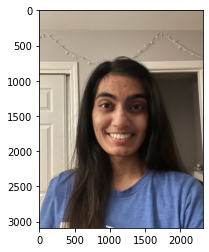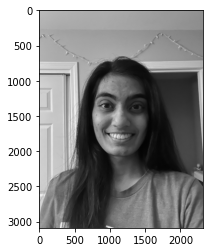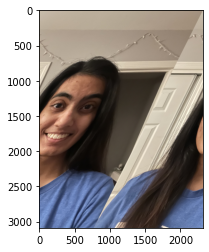In :
###########
# IMPORTS #
###########

import numpy as np
import cv2
from matplotlib import pyplot as plt

In :
########
########

# NOTE: OpenCV's image shower tends to crash my jupyter notebook so
#       I'm just using pyplot to show the image in line
#       (which also requires me to switch the color channel order)

rgb_orig = cv2.cvtColor(original, cv2.COLOR_BGR2RGB)
plt.imshow(rgb_orig)
plt.show()## Please see sections below each transformation for explanation

In :
#############
# GREYSCALE #
#############

def avg_grey_scale(img):
new_img = np.zeros(img.shape, dtype=np.uint8)
for x in range(img.shape):
for y in range(img.shape):
avg = [int(sum(img[x,y])/3) for i in range(3)]
# assign all three color channels in new img to avg of color values in each pixel
new_img[x,y] = avg
return new_img

grey_scale = avg_grey_scale(rgb_orig)

bgr_grey = cv2.cvtColor(grey_scale, cv2.COLOR_RGB2BGR)
cv2.imwrite("./greyscale.jpg", bgr_grey)

plt.imshow(grey_scale)
plt.show()Converting to Greyscale

I converted the image to greyscale by iterating through the pixels in the original image, computing the average over the three color channels, and then assigning each of the channels that value. To preserve the original image, I assigned the new pixel values into a new numpy array of the same shape.

In :
########
# TILT #
########

def tilt(img):
new_img = np.zeros(img.shape, dtype=np.uint8)
for x in range(img.shape):
arr = img[x]
new_x = [arr[(i+add)%len(arr)] for i in range(len(arr))]
new_img[x] = new_x
return new_img

tilted = tilt(rgb_orig)

bgr_tilt = cv2.cvtColor(tilted, cv2.COLOR_RGB2BGR)
cv2.imwrite("./tilted.jpg", bgr_tilt)

plt.imshow(tilted)
plt.show()Tilting the Image

I decided to try and tilt my original image for my next transformation. The way I did this was to iterate through the rows of the image and offset the pixels by more and more as I went through the image. In other words, I started the row later and later as I went lower down the image. To avoid any null pointer exceptions, I took the mod of the index (to not go over the length of the row) but that led to the previous parts of the image appearing later. I am not sure how to tilt the image such that the image stays within frame and there are no repeated sections of the image.